Publicité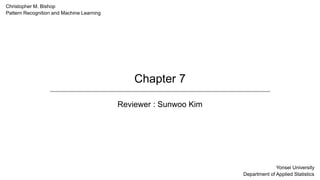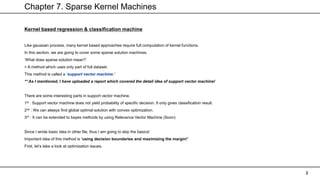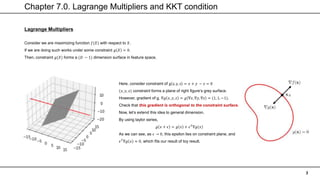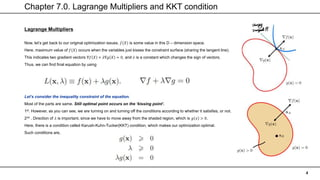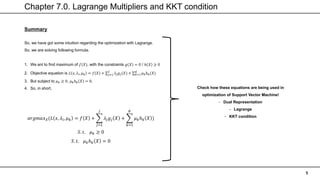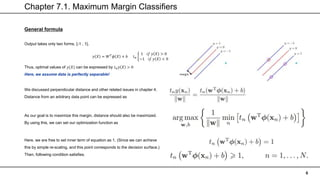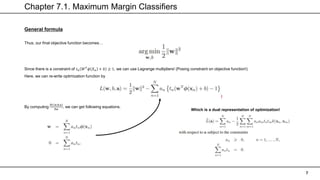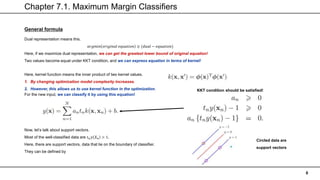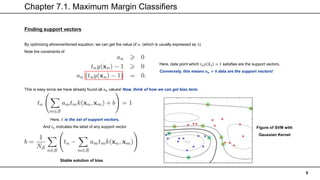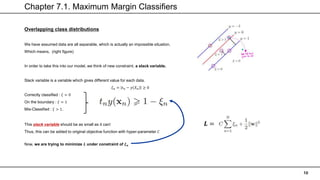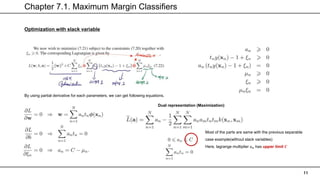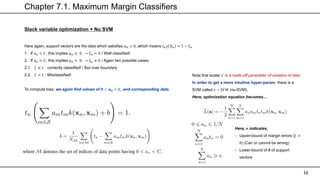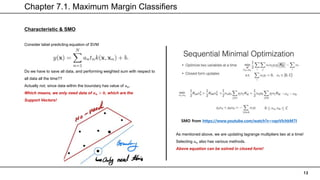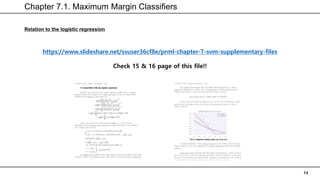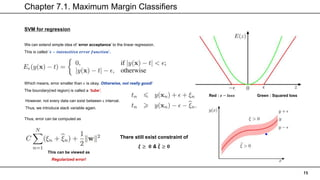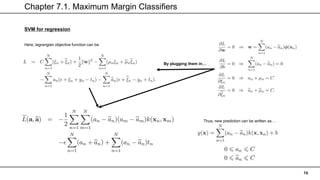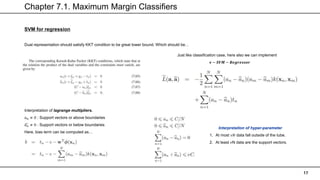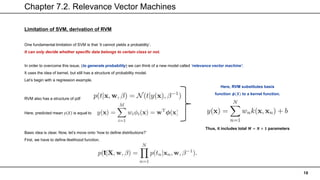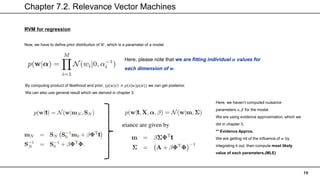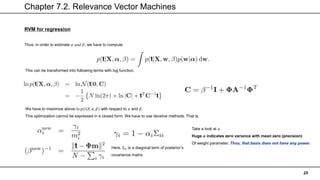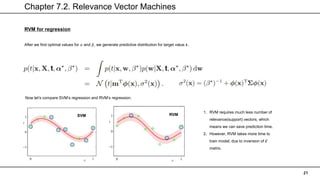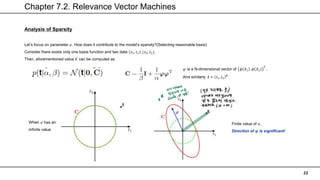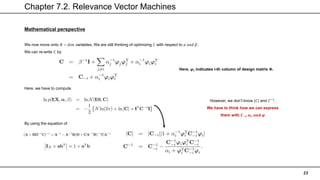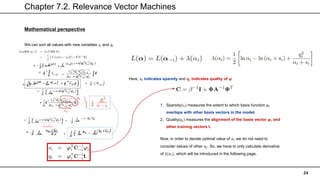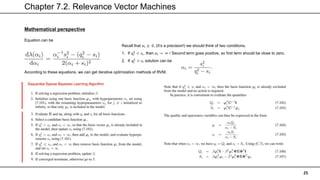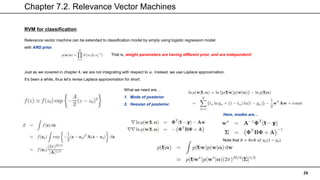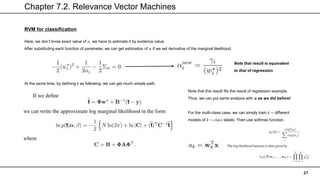1 sur 27
Publicité

### PRML Chapter 7

1. Chapter 7 Reviewer : Sunwoo Kim Christopher M. Bishop Pattern Recognition and Machine Learning Yonsei University Department of Applied Statistics
2. Chapter 7. Sparse Kernel Machines 2 Kernel based regression & classification machine Like gaussian process, many kernel based approaches require full computation of kernel functions. In this section, we are going to cover some sparse solution machines. ‘What does sparse solution mean?’ = A method which uses only part of full dataset. This method is called a ‘support vector machine.’ ** As I mentioned, I have uploaded a report which covered the detail idea of support vector machine! There are some interesting parts in support vector machine. 1st : Support vector machine does not yield probability of specific decision. It only gives classification result. 2nd : We can always find global optimal solution with convex optimization. 3rd : It can be extended to bayes methods by using Relevance Vector Machine (Soon) Since I wrote basic idea in other file, thus I am going to skip the basics! Important idea of this method is ‘using decision boundaries and maximizing the margin!’ First, let’s take a look at optimization issues.
3. Chapter 7.0. Lagrange Multipliers and KKT condition 3 Lagrange Multipliers Consider we are maximizing function 𝑓(𝑋) with respect to 𝑋. If we are doing such works under some constraint 𝑔 𝑋 = 0. Then, constraint 𝑔(𝑋) forms a (𝐷 − 1) dimension surface in feature space. Here, consider constraint of g x, y, z = 𝑥 + 𝑦 − 𝑧 = 0 (𝑥, 𝑦, 𝑧) constraint forms a plane of right figure’s grey surface. However, gradient of g, ∇𝑔 𝑥, 𝑦, 𝑧 = 𝑔 ∇𝑥, ∇𝑦, ∇𝑧 = (1, 1, −1). Check that this gradient is orthogonal to the constraint surface. Now, let’s extend this idea to general dimension. By using taylor series, 𝑔 𝑥 + 𝜖 = 𝑔 𝑥 + 𝜖𝑇 ∇𝑔(𝑥) As we can see, as 𝜖 → 0, this epsilon lies on constraint plane, and 𝜖𝑇 ∇𝑔 𝑥 ≈ 0, which fits our result of toy result.
4. Chapter 7.0. Lagrange Multipliers and KKT condition 4 Lagrange Multipliers Now, let’s get back to our original optimization issues. 𝑓(𝑋) is some value in this D – dimension space. Here, maximum value of 𝑓(𝑋) occurs when the variables just kisses the constraint surface (sharing the tangent line). This indicates two gradient vectors ∇𝑓 𝑋 + 𝜆∇𝑔 𝑋 = 0, and 𝜆 is a constant which changes the sign of vectors. Thus, we can find final equation by using Let’s consider the inequality constraint of the equation. Most of the parts are same. Still optimal point occurs on the ‘kissing point’. 1st. However, as you can see, we are turning on and turning off the conditions according to whether it satisfies, or not. 2nd . Direction of 𝜆 is important, since we have to move away from the shaded region, which is 𝑔 𝑥 > 0. Here, there is a condition called Karush-Kuhn-Tucker(KKT) condition, which makes our optimization optimal. Such conditions are,
5. Chapter 7.0. Lagrange Multipliers and KKT condition 5 Summary So, we have got some intuition regarding the optimization with Lagrange. So, we are solving following formula. 1. We ant to find maximum of 𝑓(𝑋), with the constraints 𝑔 𝑋 = 0 / ℎ 𝑋 ≥ 0 2. Objective equation is 𝐿 𝑥, 𝜆𝑖, 𝜇𝑘 = 𝑓 𝑋 + 𝑗=1 𝐽 𝜆𝑗𝑔𝑗(𝑋) + 𝑘=1 𝐾 𝜇𝑘ℎ𝑘(𝑋) 3. But subject to 𝜇𝑘 ≥ 0, 𝜇𝑘ℎ𝑘 𝑋 = 0. 4. So, in short, 𝑎𝑟𝑔𝑚𝑎𝑥𝑋(𝐿 𝑥, 𝜆𝑖, 𝜇𝑘 = 𝑓 𝑋 + 𝑗=1 𝐽 𝜆𝑗𝑔𝑗 𝑋 + 𝑘=1 𝐾 𝜇𝑘ℎ𝑘 𝑋 ) 𝑆. 𝑡. 𝜇𝑘 ≥ 0 𝑆. 𝑡. 𝜇𝑘ℎ𝑘 𝑋 = 0 Check how these equations are being used in optimization of Support Vector Machine! - Dual Representation - Lagrange - KKT condition
6. Chapter 7.1. Maximum Margin Classifiers 6 General formula Output takes only two forms, {-1 , 1}. 𝑦 𝑋 = 𝑊𝑇 𝜙 𝑋 + 𝑏 t𝑛 1 𝑖𝑓 𝑦 𝑋 > 0 −1 𝑖𝑓 𝑦 𝑋 < 0 Thus, optimal values of 𝑦(𝑋) can be expressed by 𝑡𝑛𝑦 𝑋 > 0 Here, we assume data is perfectly separable! We discussed perpendicular distance and other related issues in chapter 4. Distance from an arbitrary data point can be expressed as As our goal is to maximize this margin, distance should also be maximized. By using this, we can set our optimization function as Here, we are free to set inner term of equation as 1. (Since we can achieve this by simple re-scaling, and this point corresponds to the decision surface.) Then, following condition satisfies.
7. Chapter 7.1. Maximum Margin Classifiers 7 General formula Thus, our final objective function becomes… Since there is a constraint of 𝑡𝑛 𝑊𝑇 𝜙 𝑋𝑛 + 𝑏 ≥ 1, we can use Lagrange multipliers! (Posing constraint on objective function!) Here, we can re-write optimization function by By computing 𝜕𝐿 𝑤,𝑏,𝑎 𝜕𝑤 , we can get following equations. Which is a dual representation of optimization!
8. Chapter 7.1. Maximum Margin Classifiers 8 General formula Here, kernel function means the inner product of two kernel values. 1. By changing optimization model complexity increases. 2. However, this allows us to use kernel function in the optimization. For the new input, we can classify it by using this equation! KKT condition should be satisfied! Now, let’s talk about support vectors. Most of the well-classified data are 𝑡𝑛𝑦 𝑋𝑛 > 1. Here, there are support vectors, data that lie on the boundary of classifier. They can be defined by Circled data are support vectors Dual representation means this. 𝑎𝑟𝑔𝑚𝑖𝑛 𝑜𝑟𝑖𝑔𝑖𝑛𝑎𝑙 𝑒𝑞𝑢𝑎𝑡𝑖𝑜𝑛 ≥ (𝑑𝑢𝑎𝑙 − 𝑒𝑞𝑢𝑎𝑡𝑖𝑜𝑛) Here, if we maximize dual representation, we can get the greatest lower bound of original equation! Two values become equal under KKT condition, and we can express equation in terms of kernel!
9. Chapter 7.1. Maximum Margin Classifiers 9 Finding support vectors By optimizing aforementioned equation, we can get the value of 𝑎, (which is usually expressed as 𝜆) Note the constraints of Here, data point which 𝑡𝑛𝑦 𝑋𝑛 = 1 satisfies are the support vectors. Conversely, this means 𝒂𝒏 ≠ 𝟎 data are the support vectors! This is easy since we have already found all 𝑎𝑛 values! Now, think of how we can get bias term. Here, 𝑆 is the set of support vectors, And 𝑡𝑛 indicates the label of any support vector Figure of SVM with Gaussian Kernel Stable solution of bias
10. Chapter 7.1. Maximum Margin Classifiers 10 Overlapping class distributions We have assumed data are all separable, which is actually an impossible situation. Which means, (right figure) In order to take this into our model, we think of new constraint, a slack variable. Slack variable is a variable which gives different value for each data. 𝜉𝑛 = 𝑡𝑛 − 𝑦 𝑋𝑛 ≥ 0 Correctly classified : 𝜉 = 0 On the boundary : 𝜉 = 1 Mis-Classified : 𝜉 > 1. This slack variable should be as small as it can! Thus, this can be added to original objective function with hyper-parameter 𝐶 Now, we are trying to minimize 𝑳 under constraint of 𝝃𝒏 𝑳 =
11. Chapter 7.1. Maximum Margin Classifiers 11 Optimization with slack variable By using partial derivative for each parameters, we can get following equations. Most of the parts are same with the previous separable case example(without slack variables) Here, lagrange multiplier 𝑎𝑛 has upper limit 𝑪 Dual representation (Maximization)
12. Chapter 7.1. Maximum Margin Classifiers 12 Slack variable optimization + Nu SVM Here again, support vectors are the data which satisfies 𝑎𝑛 > 0, which means 𝑡𝑛𝑦 𝑋𝑛 = 1 − 𝜉𝑛 1. If 𝑎𝑛 < 𝐶, this implies 𝜇𝑛 ≥ 0, → 𝜉𝑛 = 0 / Well classified! 2. If 𝑎𝑛 = 𝐶, this implies 𝜇𝑛 = 0, → 𝜉𝑛 ≠ 0 / Again two possible cases. 2.1. 𝜉 ≤ 1 : correctly classified! / But over boundary 2.2. 𝜉 > 1 : Misclassified! To compute bias, we again find values of 𝟎 < 𝒂𝒏 < 𝑪, and corresponding data. Note that scalar 𝐶 is a trade-off parameter of violation of data In order to get a more intuitive hyper-param, there is a SVM called 𝜈 − 𝑆𝑉𝑀 (nu-SVM). Here, optimization equation becomes… Here, 𝝂 indicates, - Upper-bound of margin errors (𝜉 > 0) (Can or cannot be wrong) - Lower-bound of # of support vectors
13. Chapter 7.1. Maximum Margin Classifiers 13 Characteristic & SMO SMO from https://www.youtube.com/watch?v=vqoVIchkM7I As mentioned above, we are updating lagrange multipliers two at a time! Selecting 𝑎𝑛 also has various methods. Above equation can be solved in closed form! Consider label predicting equation of SVM Do we have to save all data, and performing weighted sum with respect to all data all the time?? Actually not, since data within the boundary has value of 𝑎𝑛. Which means, we only need data of 𝒂𝒏 > 𝟎, which are the Support Vectors!
14. Chapter 7.1. Maximum Margin Classifiers 14 Relation to the logistic regression https://www.slideshare.net/ssuser36cf8e/prml-chapter-7-svm-supplementary-files Check 15 & 16 page of this file!!
15. Chapter 7.1. Maximum Margin Classifiers 15 SVM for regression We can extend simple idea of ‘error acceptance’ to the linear regression. This is called ′𝝐 − 𝒊𝒏𝒕𝒆𝒏𝒔𝒊𝒕𝒊𝒗𝒆 𝒆𝒓𝒓𝒐𝒓 𝒇𝒖𝒏𝒄𝒕𝒊𝒐𝒏′. Red : 𝝐 − 𝒍𝒐𝒔𝒔 Green : Squared loss Which means, error smaller than 𝜖 is okay. Otherwise, not really good! The boundary(red region) is called a ‘tube’. However, not every data can exist between ϵ interval. Thus, we introduce slack variable again. Thus, error can be computed as This can be viewed as Regularized error! There still exist constraint of 𝝃 ≥ 𝟎 & 𝝃 ≥ 𝟎
16. Chapter 7.1. Maximum Margin Classifiers 16 SVM for regression Here, lagrangian objective function can be By plugging them in… Thus, new prediction can be written as…
17. Chapter 7.1. Maximum Margin Classifiers 17 SVM for regression Dual representation should satisfy KKT condition to be great lower bound. Which should be… Interpretation of lagrange multipliers. 𝑎𝑛 ≠ 0 : Support vectors or above boundaries 𝑎𝑛 ≠ 0 : Support vectors or below boundaries Here, bias term can be computed as… Just like classification case, here also we can implement 𝝂 − 𝑺𝑽𝑴 − 𝑹𝒆𝒈𝒓𝒆𝒔𝒔𝒐𝒓 Interpretation of hyper-parameter 1. At most 𝜈𝑁 data fall outside of the tube. 2. At least 𝜈N data are the support vectors.
18. Chapter 7.2. Relevance Vector Machines 18 Limitation of SVM, derivation of RVM One fundamental limitation of SVM is that ‘it cannot yields a probability’. It can only decide whether specific data belongs to certain class or not. In order to overcome this issue, (to generate probability) we can think of a new model called ‘relevance vector machine’. It uses the idea of kernel, but still has a structure of probability model. Let’s begin with a regression example. RVM also has a structure of pdf Here, predicted mean 𝑦 𝑋 is equal to Here, RVM substitutes basis function 𝝓(𝑿) to a kernel function. Thus, it includes total 𝑴 = 𝑵 + 𝟏 parameters Basic idea is clear. Now, let’s move onto ‘how to define distributions?’ First, we have to define likelihood function.
19. Chapter 7.2. Relevance Vector Machines 19 RVM for regression Now, we have to define prior distribution of 𝑊, which is a parameter of a model. Here, please note that we are fitting individual 𝜶 values for each dimension of 𝒘. By computing product of likelihood and prior, (𝑝 𝑤 𝑥 ∝ 𝑝 𝑥 𝑤 𝑝(𝑤)) we can get posterior. We can also use general result which we derived in chapter 3. Here, we haven’t computed nuisance parameters 𝛼, 𝛽 for the model. We are using evidence approximation, which we did in chapter 3. ** Evidence Approx. We are getting rid of the influence of 𝑤 by integrating it out, then compute most likely value of each parameters.(MLE)
20. Chapter 7.2. Relevance Vector Machines 20 RVM for regression Thus, in order to estimate 𝛼 𝑎𝑛𝑑 𝛽, we have to compute This can be transformed into following terms with log function. We have to maximize above ln 𝑝(𝑡|𝑋, 𝛼, 𝛽) with respect to 𝛼 and 𝛽. This optimization cannot be expressed in a closed form. We have to use iterative methods. That is, Here, Σ𝑖𝑖 is a diagonal term of posterior’s covariance matrix Take a look at 𝛼. Huge 𝜶 indicates zero variance with mean zero (precision) Of weight parameter. Thus, that basis does not have any power.
21. Chapter 7.2. Relevance Vector Machines 21 RVM for regression After we find optimal values for 𝛼 and 𝛽, we generate predictive distribution for target value 𝒕. Now let’s compare SVM’s regression and RVM’s regression. SVM RVM 1. RVM requires much less number of relevance(support) vectors, which means we can save prediction time. 2. However, RVM takes more time to train model, due to inversion of 𝑪 matrix.
22. Chapter 7.2. Relevance Vector Machines 22 Analysis of Sparsity Let’s focus on parameter 𝛼. How does it contribute to the model’s sparsity?(Selecting reasonable basis) Consider there exists only one basis function and two data 𝑥1, 𝑡1 , (𝑥2, 𝑡2). Then, aforementioned value 𝑪 can be computed as 𝜑 is a N-dimensional vector of 𝜙 𝑋1 , 𝜙 𝑋2 𝑇 . And similarly 𝒕 = 𝑡1, 𝑡2 𝑻 When 𝛼 has an infinite value Finite value of 𝛼. Direction of 𝝋 is significant!
23. Chapter 7.2. Relevance Vector Machines 23 Mathematical perspective We now move onto 𝑁 − 𝑑𝑖𝑚 variables. We are still thinking of optimizing 𝐶 with respect to 𝛼 𝑎𝑛𝑑 𝛽. We can re-write 𝐶 by Here, 𝝋𝒊 indicates i-th column of design matrix 𝚽. Here, we have to compute However, we don’t know |𝐶| and 𝐶−1 . We have to think how we can express them with 𝑪−𝒊, 𝜶𝒊, 𝒂𝒏𝒅 𝝋 By using the equation of
24. Chapter 7.2. Relevance Vector Machines 24 Mathematical perspective We can sort all values with new variables 𝑠𝑖 and 𝑞𝑖 Here, 𝒔𝒊 indicates sparsity and 𝒒𝒊 indicates quality of 𝝋 1. Sparsity(𝑠𝑖) measures the extent to which basis function 𝜑𝑖 overlaps with other basis vectors in the model. 2. Quality(𝑞𝑖) measures the alignment of the basis vector 𝝋𝒊 and other training vectors t. Now, in order to decide optimal value of 𝛼𝑖 we do not need to consider values of other 𝛼𝑗. So, we have to only calculate derivative of 𝜆(𝛼𝑖), which will be introduced in the following page.
25. Chapter 7.2. Relevance Vector Machines 25 Mathematical perspective Equation can be Recall that 𝛼𝑖 ≥ 0, (It’s a precision!) we should think of two conditions. 1. If 𝑞𝑖 2 < 𝑠𝑖, then 𝛼𝑖 → ∞ / Second term goes positive, so first term should be close to zero. 2. If 𝑞𝑖 2 > 𝑠𝑖 solution can be According to these equations, we can get iterative optimization methods of RVM.
26. Chapter 7.2. Relevance Vector Machines 26 RVM for classification Relevance vector machine can be extended to classification model by simply using logistic regression model with ARD prior. Just as we covered in chapter 4, we are not integrating with respect to 𝑤. Instead, we use Laplace approximation. It’s been a while, thus let’s revise Laplace approximation for short. That is, weight parameters are having different prior, and are independent! What we need are… 1. Mode of posterior 2. Hessian of posterior. Here, modes are… Note that 𝐵 = 𝑁𝑥𝑁 𝑜𝑓 𝑦𝑛(1 − 𝑦𝑛)
27. Chapter 7.2. Relevance Vector Machines 27 RVM for classification Here, we don’t know exact value of 𝛼, we have to estimate it by evidence value. After substituting each function of parameter, we can get estimation of 𝛼 if we set derivative of the marginal likelihood. Note that result is equivalent to that of regression At the same time, by defining 𝒕 as following, we can get much simple path. Note that this result fits the result of regression example. Thus, we can put same analysis with 𝜶 as we did before! For the multi-class case, we can simply train 𝑘 − different models of 𝑘 − 𝑐𝑙𝑎𝑠𝑠 labels. Then use softmax function.
Publicité